Test: Fractions- 1

# Test: Fractions- 1

Test Description

## 10 Questions MCQ Test Mathematics for Class 3 | Test: Fractions- 1

Test: Fractions- 1 for Class 3 2023 is part of Mathematics for Class 3 preparation. The Test: Fractions- 1 questions and answers have been prepared according to the Class 3 exam syllabus.The Test: Fractions- 1 MCQs are made for Class 3 2023 Exam. Find important definitions, questions, notes, meanings, examples, exercises, MCQs and online tests for Test: Fractions- 1 below.
Solutions of Test: Fractions- 1 questions in English are available as part of our Mathematics for Class 3 for Class 3 & Test: Fractions- 1 solutions in Hindi for Mathematics for Class 3 course. Download more important topics, notes, lectures and mock test series for Class 3 Exam by signing up for free. Attempt Test: Fractions- 1 | 10 questions in 15 minutes | Mock test for Class 3 preparation | Free important questions MCQ to study Mathematics for Class 3 for Class 3 Exam | Download free PDF with solutions
 1 Crore+ students have signed up on EduRev. Have you?
Test: Fractions- 1 - Question 1

### What fraction of the figure is shaded?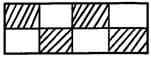Detailed Solution for Test: Fractions- 1 - Question 1

Count the total number of parts = 8
The number of parts shaded red = 4
Fraction of the figure shaded = 4 / 8 = 1 / 2.

Test: Fractions- 1 - Question 2

### How many equal parts does the figure below have?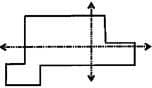Detailed Solution for Test: Fractions- 1 - Question 2

The dotted lines divide the shape in 4 parts.
However none of the parts are equal.
Hence there are 0 equal parts in the figure.

Test: Fractions- 1 - Question 3

### How many equal parts does the shape below have?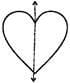Detailed Solution for Test: Fractions- 1 - Question 3

The dotted line divided the heart shape in 2 equal parts.

Test: Fractions- 1 - Question 4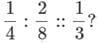Detailed Solution for Test: Fractions- 1 - Question 4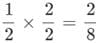Similarly,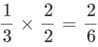Test: Fractions- 1 - Question 5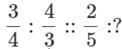Detailed Solution for Test: Fractions- 1 - Question 5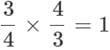Similarly,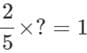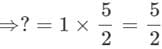Test: Fractions- 1 - Question 6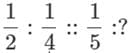Detailed Solution for Test: Fractions- 1 - Question 6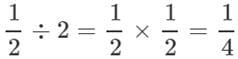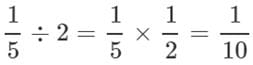Test: Fractions- 1 - Question 7

Which fraction is odd one out?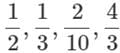Detailed Solution for Test: Fractions- 1 - Question 7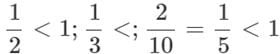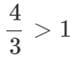So,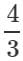is odd one out.

Test: Fractions- 1 - Question 8

Ravi collected 50 stamps and Surya collected one-fifth of them. How many stamps does Ravi and Surya have in all?

Detailed Solution for Test: Fractions- 1 - Question 8

One-fifth of 50 = 1 / 5 x 50 = 10.
Surya has 10 stamps.
Total stamps w/ Ravi and Surya = 50 + 10 = 60 stamps.

Test: Fractions- 1 - Question 9

The Venn diagram below shows the favourite drink for 20 students.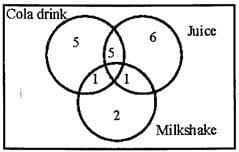Q. What fraction of students like juice and not milkshake?

Detailed Solution for Test: Fractions- 1 - Question 9

Total no. of students = 20
No. of students who like juice and not milk shake = 6 + 5 = 11
Fraction students who like juice and not milk shake =11 / 20

Test: Fractions- 1 - Question 10

The Venn diagram below shows the favourite sports for 20 children.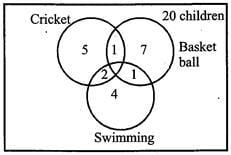Q. What fraction of students like cricket?

Detailed Solution for Test: Fractions- 1 - Question 10

Total no. of students = 20
No. of students who like cricket = 5 + 2 + 1 = 8
Fraction of students who like cricket = 8 / 20 = 25.

## Mathematics for Class 3

15 videos|53 docs|23 tests
 Use Code STAYHOME200 and get INR 200 additional OFF Use Coupon Code
Information about Test: Fractions- 1 Page
In this test you can find the Exam questions for Test: Fractions- 1 solved & explained in the simplest way possible. Besides giving Questions and answers for Test: Fractions- 1, EduRev gives you an ample number of Online tests for practice

## Mathematics for Class 3

15 videos|53 docs|23 tests